# Lagrange equations (in mechanics)

(diff) ← Older revision | Latest revision (diff) | Newer revision → (diff)

Ordinary second-order differential equations which describe the motions of mechanical systems under the action of forces applied to them. The equations were established by J.L. Lagrange  in two forms: Lagrange's equations of the first kind, or equations in Cartesian coordinates with undetermined Lagrange multipliers, and of the second kind, or equations in generalized Lagrange coordinates.

Lagrange's equations of the first kind describe motions of both holonomic systems, constrained only by geometrical relations of the form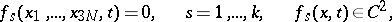(1)

and non-holonomic systems, on which one imposes, as well as relations (1), kinematic relations of the form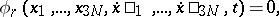(2)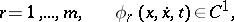whereand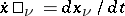are the Cartesian coordinates and the velocities of the points,is the number of points of the system,is the time, and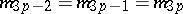is the mass of the-th point, which has coordinates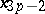,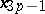,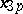.

The relations (1) and (2) are assumed to be independent, that is, the ranks of the matrices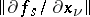and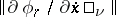are equal toand, respectively. Lagrange's equations of the first kind have the form(3)

where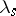andare undetermined Lagrange multipliers, proportional to the reactions of the constraints,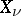are the projections on the coordinate axes of the given active forces, and the force, acting on the-th point, has the projections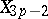,,;.

To the differential equations (3) one must adjoin the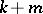equations (1) and (2), as a result of which one obtains a system ofequations in the same number of variables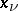,,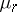. In practice, Lagrange's equations of the first kind are usually applied to systems with a small number of unknowns.

Lagrange's equations of the second kind describe only motions of holonomic systems restricted by constraints of the form (1). By introducing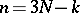independent generalized Lagrange coordinates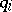, by means of which any possible position of the system can be obtained for certain values of thefrom the equalities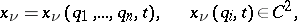(4)

that convert equations (1) into identities, one can establish for everya one-to-one correspondence between the possible positions of the system and the points of some region of the-dimensional configuration space. In the case of stationary constraints (1) it is always possible to choose the variablesso that the timedoes not occur in (4). Also, by means of (4) one can write down expressions for the sum of the elementary works of all the active forcescorresponding to all possible displacements of the system:and the kinetic energy of the system: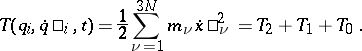Hereis the generalized force corresponding to the coordinate, the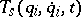are homogeneous forms of degreein the generalized velocities, and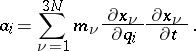In the case of stationary constraints.

Lagrange's equations of the second kind have the form(5)

Equations (5) form a system ofordinary second-order differential equations with unknowns. Their form is invariant with respect to the choice of Lagrange coordinates. This system of equations of motion has least possible order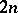. In this respect, and also in the absence of reactions of the constraints in (5), equations (5) have a great advantage compared to Lagrange's equations of the first kind (3). After integrating (5) one can determine the reactions of the constraints from the equations that express Newton's second law for the points of the system (cf. also Newton laws of mechanics).

In the case of potential generalized forces, when there is a force functionsuch that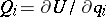,, equations (5) take the form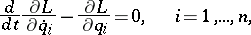(6)

where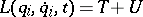is called the Lagrange function (formerly, the kinetic potential).

If, or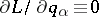, then equations (6) have a generalized energy integralor a cyclic integralcorresponding to the cyclic coordinate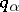.

How to Cite This Entry:
Lagrange equations (in mechanics). Encyclopedia of Mathematics. URL: http://encyclopediaofmath.org/index.php?title=Lagrange_equations_(in_mechanics)&oldid=17218
This article was adapted from an original article by V.V. Rumyantsev (originator), which appeared in Encyclopedia of Mathematics - ISBN 1402006098. See original article